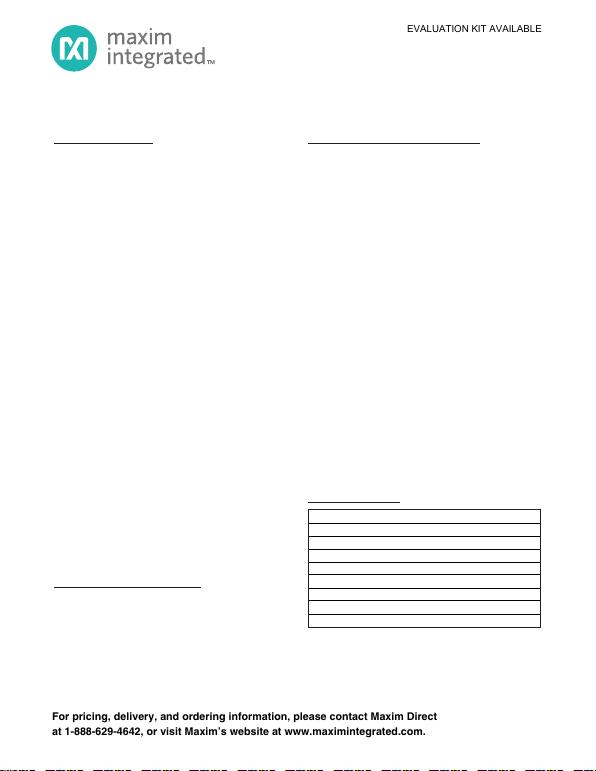AVAILABLE
EVALUATION KIT AVAILABLE
Functional Diagrams
Pin Configurations appear at end of data sheet.
Functional Diagrams continued at end of data sheet.
UCSP is a trademark of Maxim Integrated Products, Inc.
at 1-888-629-4642, or visit Maxim’s website at www.maximintegrated.com.
General Description
The MAX15004A/B/MAX15005A/B high-performance,
current-mode PWM controllers operate at an automo-
tive input voltage range from 4.5V to 40V (load dump).
The input voltage can go down as low as 2.5V after
startup if VCC is supplied by an external bias voltage.
The controllers integrate all the building blocks neces-
sary for implementing fixed-frequency isolated/noniso-
lated power supplies. The general-purpose boost,
flyback, forward, and SEPIC converters can be
designed with ease around the MAX15004/MAX15005.
The current-mode control architecture offers excellent
line-transient response and cycle-by-cycle current limit
while simplifying the frequency compensation.
Programmable slope compensation simplifies the
design further. A fast 60ns current-limit response time,
low 300mV current-limit threshold makes the controllers
suitable for high-efficiency, high-frequency DC-DC con-
verters. The devices include an internal error amplifier
and 1% accurate reference to facilitate the primary-side
regulated, single-ended flyback converter or nonisolat-
ed converters.
An external resistor and capacitor network programs
the switching frequency from 15kHz to 500kHz (1MHz for
the MAX15005A/B). The MAX15004A/B/MAX15005A/B
provide a SYNC input for synchronization to an external
clock. The maximum FET-driver duty cycle for the
MAX15004A/B is 50%. The maximum duty cycle can be
set on the MAX15005A/B by selecting the right combi-
nation of RT and CT.
The input undervoltage lockout (ON/OFF) programs the
input-supply startup voltage and can be used to shut-
down the converter to reduce the total shutdown cur-
rent down to 10µA. Protection features include
cycle-by-cycle and hiccup current limit, output overvolt-
age protection, and thermal shutdown.
The MAX15004A/B/MAX15005A/B are available in
space-saving 16-pin TSSOP and thermally enhanced
16-pin TSSOP-EP packages. All devices operate over
the -40°C to +125°C automotive temperature range.
Applications
Automotive
Vacuum Fluorescent Display (VFD) Power
Supply
Isolated Flyback, Forward, Nonisolated SEPIC,
Boost Converters
Features
Wide 4.5V to 40V Operating Input Voltage Range
Operates Down to 2.5V (with Bootstrapped VCC
Bias)
Current-Mode Control
Low 300mV, 5% Accurate Current-Limit Threshold
Voltage
Internal Error Amplifier with 1% Accurate Reference
RC Programmable 4% Accurate Switching
Frequency
Switching Frequency Adjustable from 15kHz to
500kHz (1MHz for the MAX15005A/B)
External Frequency Synchronization
Maximum Duty Cycle
Programmable Slope Compensation
10µA Shutdown Current
Cycle-by-Cycle and Hiccup Current-Limit
Protection
Overvoltage and Thermal Shutdown Protection
-40°C to +125°C Automotive Temperature Range
16-Pin TSSOP or 16-Pin Thermally Enhanced
TSSOP-EP Packages
AEC-Q100 Qualified
4.5V to 40V Input Automotive
Flyback/Boost/SEPIC Power-Supply Controllers
PART PIN-PACKAGE MAX DUTY CYCLE
MAX15004AAUE+ 16 TSSOP-EP* 50%
MAX15004AAUE/V+ 16 TSSOP-EP* 50%
MAX15004BAUE+ 16 TSSOP 50%
MAX15004BAUE/V+ 16 TSSOP 50%
MAX15005AAUE+ 16 TSSOP-EP* Programmable
MAX15005AAUE/V+ 16 TSSOP-EP* Programmable
MAX15005BAUE+ 16 TSSOP Programmable
MAX15005BAUE/V+ 16 TSSOP Programmable
Ordering Information
Note: All devices are specified over the -40°C to +125°C
temperature range.
+
/V denotes an automotive qualified part.
*
Pin Configurations appear at end of data sheet.
MAX15004A/B/MAX15005A/B
19-0723; Rev 3; 1/11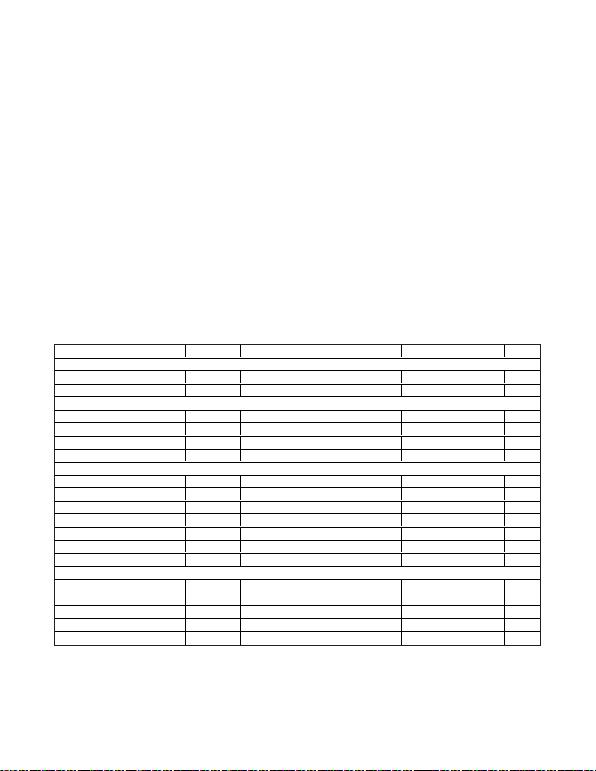4.5V to 40V Input Automotive
Flyback/Boost/SEPIC Power-Supply Controllers
ABSOLUTE MAXIMUM RATINGS
ELECTRICAL CHARACTERISTICS
(VIN = 14V, CIN = 0.1μF, CVCC = 0.1μF // 1μF, CREG5 = 1μF, VON/OFF = 5V, CSS = 0.01μF, CSLOPE = 100pF, RT = 13.7kΩ, CT =
560pF, VSYNC = VOVI = VFB = VCS = 0V, COMP = unconnected, OUT = unconnected. TA= TJ= -40°C to +125°C, unless otherwise
noted. Typical values are at TA = +25°C. All voltages are referenced to PGND, unless otherwise noted.) (Note 1) (Figure 5)
Stresses beyond those listed under “Absolute Maximum Ratings” may cause permanent damage to the device. These are stress ratings only, and functional
operation of the device at these or any other conditions beyond those indicated in the operational sections of the specifications is not implied. Exposure to
absolute maximum rating conditions for extended periods may affect device reliability.
IN to SGND.............................................................-0.3V to +45V
IN to PGND.............................................................-0.3V to +45V
ON/OFF to SGND ........................................-0.3V to (VIN + 0.3V)
OVI, SLOPE, RTCT, SYNC, SS, FB, COMP,
CS to SGND.........................................-0.3V to (VREG5 + 0.3V)
VCC to PGND..........................................................-0.3V to +12V
REG5 to SGND .........................................................-0.3V to +6V
OUT to PGND.............................................-0.3V to (VCC + 0.3V)
SGND to PGND .....................................................-0.3V to +0.3V
VCC Sink Current (clamped mode).....................................35mA
OUT Current (< 10μs transient) ..........................................±1.5A
Continuous Power Dissipation* (TA= +70°C)
16-Pin TSSOP-EP (derate 21.3mW/°C
above +70°C)..............................................................1702mW
16-Pin TSSOP (derate 9.4mW/°C above +70°C) ..........754mW
Operating Junction Temperature Range...........-40°C to +125°C
Junction Temperature......................................................+150°C
Storage Temperature Range .............................-60°C to +150°C
Soldering Temperature (reflow) .......................................+260°C
*
As per JEDEC51 Standard, Multilayer Board.
PARAMETER SYMBOL CONDITIONS MIN TYP MAX UNITS
POWER SUPPLY
Input Supply Range VIN 4.5 40.0 V
Operating Supply Current IQVIN = 40V, fOSC = 150kHz 2 3.1 mA
ON/OFF CONTROL
Input-Voltage Threshold VON VON/OFF rising 1.05 1.23 1.40 V
Input-Voltage Hysteresis VHYST-ON 75 mV
Input Bias Current IB-ON/OFF VON/OFF = 40V 0.5 μA
Shutdown Current ISHDN VON/OFF = 0V 10 20 μA
INTERNAL 7.4V LDO (VCC)
Output (VCC) Voltage Set Point VVCC IVCC = 0 to 20mA (sourcing) 7.15 7.4 7.60 V
Line Regulation VIN = 8V to 40V 1 mV/V
UVLO Threshold Voltage VUVLO-VCC VCC rising 3.15 3.5 3.75 V
UVLO Hysteresis VHYST-UVLO 500 mV
Dropout Voltage VIN = 4.5V, IVCC = 20mA (sourcing) 0.25 0.5 V
Output Current Limit IVCC-ILIM IVCC sourcing 45 mA
Internal Clamp Voltage VVCC-CLAMP IVCC = 30mA (sinking) 10.0 10.4 10.8 V
INTERNAL 5V LDO (REG5)
Output (REG5) Voltage Set Point VREG5 VCC = 7.5V, IREG5 = 0 to 15mA (sourcing) 4.75 4.95 5.05 V
Line Regulation VCC = 5.5V to 10V 2 mV/V
Dropout Voltage VCC = 4.5V, IREG5 = 15mA (sourcing) 0.25 0.5 V
Output Current Limit IREG5-ILIM IREG5 sourcing 32 mA
MAX15004A/B/MAX15005A/B
2
Maxim Integrated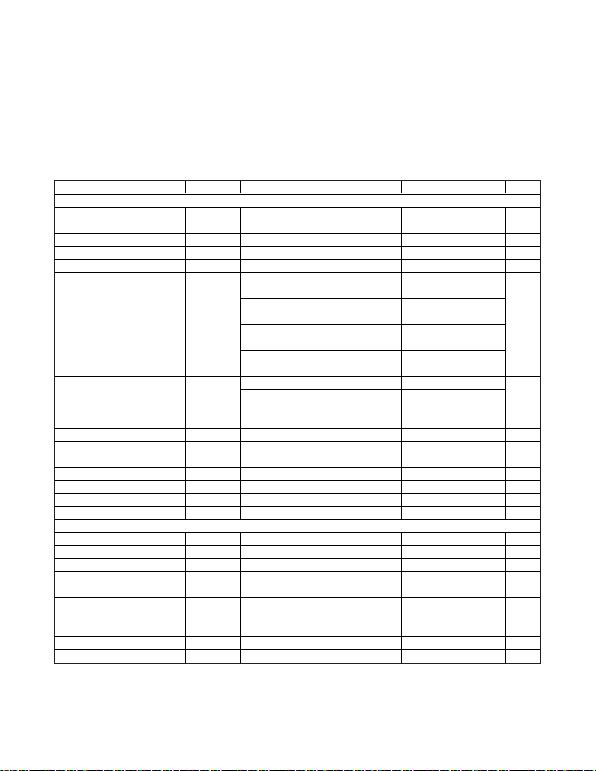4.5V to 40V Input Automotive
Flyback/Boost/SEPIC Power-Supply Controllers
PARAMETER SYMBOL CONDITIONS MIN TYP MAX UNITS
OSCILLATOR (RTCT)
Oscillator Frequency Range fOSC fOSC = 2 x fOUT for MAX15004A/B,
fOSC = fOUT for MAX15005A/B 15 1000 kHz
RTCT Peak Trip Level VTH,RTCT 0.55 x VREG5 V
RTCT Valley Trip Level VTL,RTCT 0.1 x VREG5 V
RTCT Discharge Current IDIS,RTCT VRTCT = 2V 1.30 1.33 1.36 mA
RT = 13.7kΩ, CT = 4.7nF,
fOSC (typ) = 18kHz -4 +4
RT = 13.7kΩ, CT = 560pF,
fOSC (typ) = 150kHz -4 +4
RT = 21kΩ, CT = 100pF,
fOSC (typ) = 500kHz -5 +5
Oscillator Frequency Accuracy
(Note 2)
RT = 7kΩ, CT = 100pF,
fOSC (typ) = 1MHz -7 +7
%
MAX15004A/B 50
Maximum PWM Duty Cycle
(Note 3) DMAX MAX15005A/B,
RT = 13.7kΩ, CT = 560pF,
fOSC (typ) = 150kHz
78.5 80 81.5 %
Minimum On-Time tON-MIN VIN = 14V 110 170 ns
SYNC Lock-In Frequency Range
(Note 4)
RT = 13.7kΩ, CT = 560pF,
fOSC (typ) = 150kHz 102 200 %fOSC
SYNC High-Level Voltage VIH-SYNC 2V
SYNC Low-Level Voltage VIL-SYNC 0.8 V
SYNC Input Current ISYNC VSYNC = 0 to 5V -0.5 +0.5 μA
SYNC Minimum Input Pulse Width 50 ns
ERROR AMPLIFIER/SOFT-START
Soft-Start Charging Current ISS VSS = 0V 8 15 21 μA
SS Reference Voltage VSS 1.215 1.228 1.240 V
SS Threshold for HICCUP Enable VSS rising 1.1 V
FB Regulation Voltage VREF-FB COMP = FB,
ICOMP = -500μA to +500μA 1.215 1.228 1.240 V
FB Input Offset Voltage VOS-FB
COMP = 0.25V to 4.5V,
ICOMP = -500μA to +500μA,
VSS = 0 to 1.5V
-5 +5 mV
FB Input Current VFB = 0 to 1.5V -300 +300 nA
COMP Sink Current ICOMP-SINK VFB = 1.5V, VCOMP = 0.25V 3 5.5 mA
ELECTRICAL CHARACTERISTICS (continued)
(VIN = 14V, CIN = 0.1μF, CVCC = 0.1μF // 1μF, CREG5 = 1μF, VON/OFF = 5V, CSS = 0.01μF, CSLOPE = 100pF, RT = 13.7kΩ, CT =
560pF, VSYNC = VOVI = VFB = VCS = 0V, COMP = unconnected, OUT = unconnected. TA= TJ= -40°C to +125°C, unless otherwise
noted. Typical values are at TA = +25°C. All voltages are referenced to PGND, unless otherwise noted.) (Note 1) (Figure 5)
MAX15004A/B/MAX15005A/B
Maxim Integrated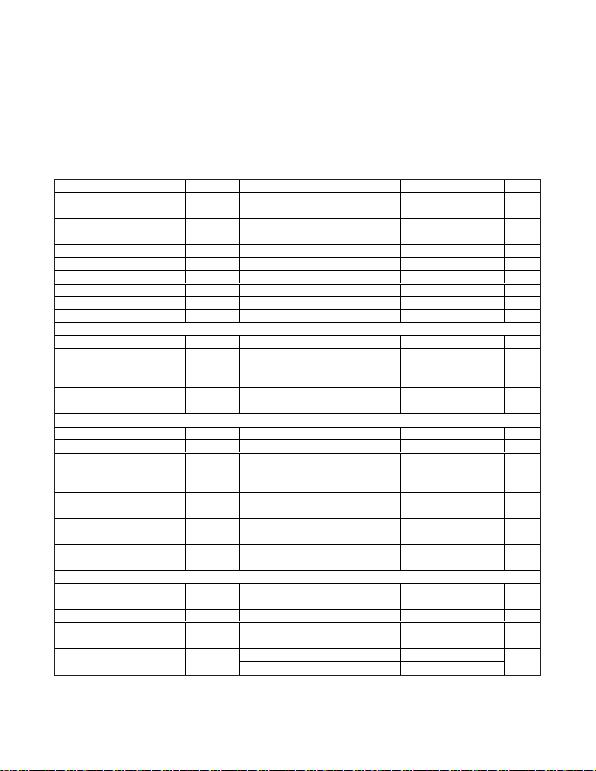4.5V to 40V Input Automotive
Flyback/Boost/SEPIC Power-Supply Controllers
ELECTRICAL CHARACTERISTICS (continued)
(VIN = 14V, CIN = 0.1μF, CVCC = 0.1μF // 1μF, CREG5 = 1μF, VON/OFF = 5V, CSS = 0.01μF, CSLOPE = 100pF, RT = 13.7kΩ, CT =
560pF, VSYNC = VOVI = VFB = VCS = 0V, COMP = unconnected, OUT = unconnected. TA= TJ= -40°C to +125°C, unless otherwise
noted. Typical values are at TA = +25°C. All voltages are referenced to PGND, unless otherwise noted.) (Note 1) (Figure 5)
PARAMETER SYMBOL CONDITIONS MIN TYP MAX UNITS
COMP Source Current ICOMP-
SOURCE VFB = 1V, VCOMP = 4.5V 1.3 2.8 mA
COMP High Voltage VOH-COMP VFB = 1V, ICOMP = 1mA (sourcing) VREG5
- 0.5
VREG5
- 0.2 V
COMP Low Voltage VOL-COMP VFB = 1.5V, ICOMP = 1mA (sinking) 0.1 0.25 V
Open-Loop Gain AEAMP 100 dB
Unity-Gain Bandwidth UGFEAMP 1.6 MHz
Phase Margin PMEAMP 75 degrees
COMP Positive Slew Rate SR+ 0.5 V/μs
COMP Negative Slew Rate SR- -0.5 V/μs
PWM COMPARATOR
Current-Sense Gain ACS-PWM ΔVCOMP/ΔVCS (Note 5) 2.85 3 3.15 V/V
PWM Propagation Delay to OUT tPD-PWM
CS = 0.15V, from VCOMP falling edge:
3V to 0.5V to OUT falling (excluding
60 ns
PWM Comparator Current-Sense
Leading-Edge Blanking Time tCS-BLANK 50 ns
CURRENT-LIMIT COMPARATOR
Current-Limit Threshold Voltage VILIM 290 305 317 mV
Current-Limit Input Bias Current IB-CS OUT= high, 0 VCS 0.3V -2 +2 μA
ILIMIT Propagation Delay to OUT tPD-ILIM
From CS rising above VILIM (50mV
overdrive) to OUT falling (excluding
60 ns
ILIM Comparator Current-Sense
Leading-Edge Blanking Time tCS-BLANK 50 ns
Number of Consecutive ILIMIT
Events to HICCUP 7
HICCUP Timeout 512 Clock
periods
SLOPE COMPENSATION (Note 6)
Slope Capacitor Charging
Current ISLOPE VSLOPE = 100mV 9.8 10.5 11.2 μA
Slope Compensation CSLOPE = 100pF 25 mV/μs
Slope Compensation Tolerance
(Note 2) CSLOPE = 100pF -4 +4 %
CSLOPE = 22pF 110
Slope Compensation Range CSLOPE = 1000pF 2.5 mV/μs
MAX15004A/B/MAX15005A/B
4
Maxim Integrated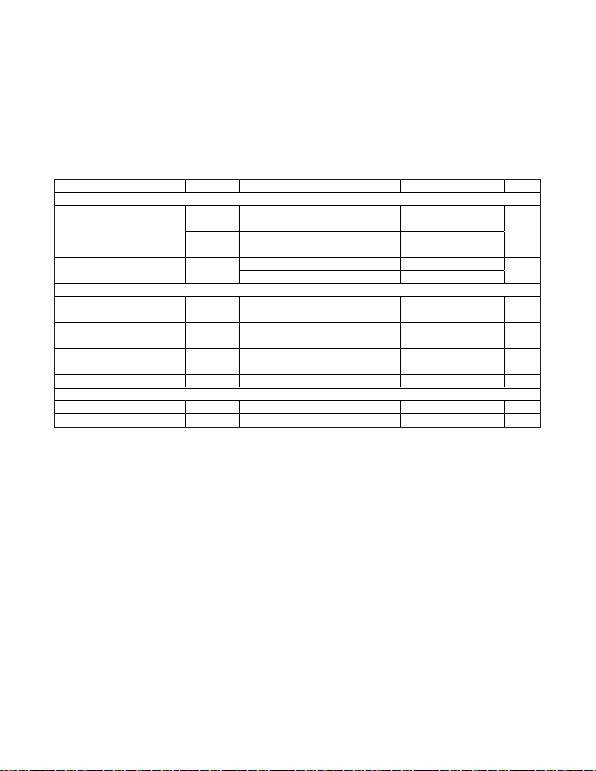4.5V to 40V Input Automotive
Flyback/Boost/SEPIC Power-Supply Controllers
ELECTRICAL CHARACTERISTICS (continued)
(VIN = 14V, CIN = 0.1μF, CVCC = 0.1μF // 1μF, CREG5 = 1μF, VON/OFF = 5V, CSS = 0.01μF, CSLOPE = 100pF, RT = 13.7kΩ, CT =
560pF, VSYNC = VOVI = VFB = VCS = 0V, COMP = unconnected, OUT = unconnected. TA= TJ= -40°C to +125°C, unless otherwise
noted. Typical values are at TA = +25°C. All voltages are referenced to PGND, unless otherwise noted.) (Note 1) (Figure 5)
PARAMETER SYMBOL CONDITIONS MIN TYP MAX UNITS
OUTPUT DRIVER
ROUT-N VCC = 8V (applied externally),
IOUT = 100mA (sinking) 1.7 3.5
Driver Output Impedance
ROUT-P VCC = 8V (applied externally),
IOUT = 100mA (sourcing) 35
Ω
COUT = 10nF, sinking 1000
Driver Peak Output Current IOUT-PEAK COUT = 10nF, sourcing 750 mA
OVERVOLTAGE COMPARATOR
Overvoltage Comparator Input
Threshold VOV-TH VOVI rising 1.20 1.228 1.26 V
Overvoltage Comparator
Hysteresis VOV-HYST 125 mV
Overvoltage Comparator Delay TDOVI From OVI rising above 1.228V to OUT
falling, with 50mV overdrive 1.6 μs
OVI Input Current IOVI VOVI = 0 to 5V -0.5 +0.5 μA
THERMAL SHUTDOWN
Shutdown Temperature TSHDN Temperature rising 160 oC
Thermal Hysteresis THYST 15 oC
Note 1: 100% production tested at +125°C. Limits over the temperature range are guaranteed by design.
Note 2: Guaranteed by design; not production tested.
Note 3: For the MAX15005A/B, DMAX depends upon the value of RT. See Figure 3 and the
Oscillator Frequency/External
Synchronization
section.
Note 4: The external SYNC pulse triggers the discharge of the oscillator ramp. See Figure 2. During external SYNC, DMAX = 50% for
the MAX15004A/B; for the MAX15005A/B, there is a shift in DMAX with fSYNC/fOSC ratio (see the
Oscillator Frequency/
External Synchronization
section).
Note 5: The parameter is measured at the trip point of latch, with 0 VCS 0.3V, and FB = COMP.
Note 6: Slope compensation = (2.5 x 10-9)/CSLOPE mV/μs. See the
Applications Information
section.
MAX15004A/B/MAX15005A/B
Maxim Integrated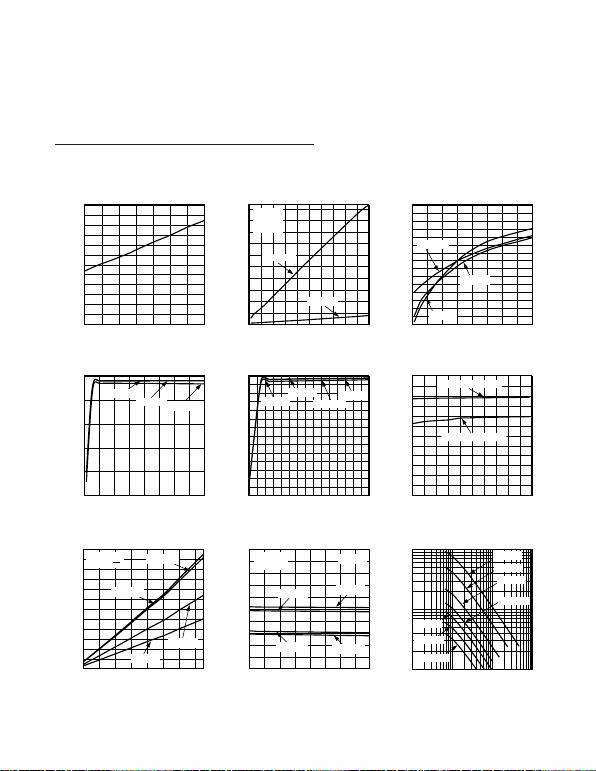4.5V to 40V Input Automotive
Flyback/Boost/SEPIC Power-Supply Controllers
Typical Operating Characteristics
(VIN = 14V, CIN = 0.1μF, CVCC = 0.1μF // 1μF, CREG5 = 1μF, VON/OFF = 5V, CSS = 0.01μF, CSLOPE = 100pF, RT = 13.7kΩ,
CT = 560pF. TA = +25°C, unless otherwise noted.)
VIN UVLO HYSTERESIS
vs. TEMPERATURE
TEMPERATURE (°C)
VIN UVLO HYSTERESIS (mV)
MAX15004 toc01
-40 -15 10 35 60 85 110 135
0
10
20
30
40
50
60
70
80
90
100
110
120
VIN SUPPLY CURRENT (ISUPPLY)
vs. OSCILLATOR FREQUENCY (fOSC)
FREQUENCY (kHz)
VIN SUPPLY CURRENT (mA)
MAX15004 toc02
10 60 110 160 210 260 310 360 410 460 510
1
4
7
10
13
16
19
22
25
28
31 MAX15005
VIN = 14V
CT = 220pF
COUT = 10nF
COUT = 0nF
SHUTDOWN SUPPLY CURRENT
vs. SUPPLY VOLTAGE
SUPPLY VOLTAGE (V)
VIN SHUTDOWN SUPPLY CURRENT (μA)
MAX15004 toc03
5 1015202530354045
5
6
7
8
9
10
11
12
13
14
15
16
17
18
19
20
TA = +135°C
TA = -40°C
TA = +25°C
VCC OUTPUT VOLTAGE
vs. VIN SUPPLY VOLTAGE
VIN SUPPLY VOLTAGE (V)
VCC OUTPUT VOLTAGE (V)
MAX15004 toc04
5 1015202530354045
5.0
5.5
6.0
6.5
7.0
7.5
IVCC = 0mA
IVCC = 1mA IVCC = 20mA
VCC CLAMP VOLTAGE
vs. VCC CURRENT SINK (IVCC)
VCC CURRENT SINK (mA)
VCC CLAMP VOLTAGE (V)
MAX15004 toc05
0 2 4 6 8 10 12 14 16 18 20 22 24 26 28 30
7.00
7.25
7.50
7.75
8.00
8.25
8.50
8.75
9.00
9.25
9.50
9.75
10.00
10.25
10.50
TA = +135°C
TA = -40°C
TA = +25°C
TA = +125°C
REG5 OUTPUT VOLTAGE
vs. VCC VOLTAGE
VCC VOLTAGE (V)
REG5 OUTPUT VOLTAGE (V)
MAX15004 toc06
5.5 6.0 6.5 7.0 7.5 8.0 8.5 9.0 9.5 10.0 10.5
4.700
4.725
4.750
4.775
4.800
4.825
4.850
4.875
4.900
4.925
4.950
4.975
5.000
IREG5 = 1mA (SOURCING)
IREG5 = 15mA (SOURCING)
REG5 DROPOUT VOLTAGE
vs. IREG5
IREG5 (mA)
REG5 LDO DROPOUT VOLTAGE (V)
MAX15004 toc07
0 2 4 6 8 10 12 14
0
0.03
0.05
0.08
0.10
0.13
0.15
0.18
0.20
0.23
0.25
0.28
0.30
TA = +135°C
TA = +25°C
TA = -40°C
TA = +125°C
VCC = 4.5
VIN = VON/OFF
OSCILLATOR FREQUENCY (fOSC)
vs. VIN SUPPLY VOLTAGE
VIN SUPPLY VOLTAGE (V)
OSCILLATOR FREQUENCY (kHz)
MAX15004 toc08
5.5 10.5 15.5 20.5 25.5 30.5 35.5 40.5 45.5
140
141
142
143
144
145
146
147
148
149
150
TA = +125°C
TA = -40°C
TA = +25°C
TA = +135°C
RT = 13.7kΩ
CT = 560pF MAX15005
OSCILLATOR FREQUENCY (fOSC)
vs. RT/CT
RT (kΩ)
OSCILLATOR FREQUENCY (kHz)
MAX15004 toc09
1 10 100 1000
10
100
1000
CT = 220pF
CT = 1500pF
CT = 1000pF
CT = 560pF
CT = 2200pF
CT = 3300pF
CT = 100pF
MAX15004A/B/MAX15005A/B
6
Maxim Integrated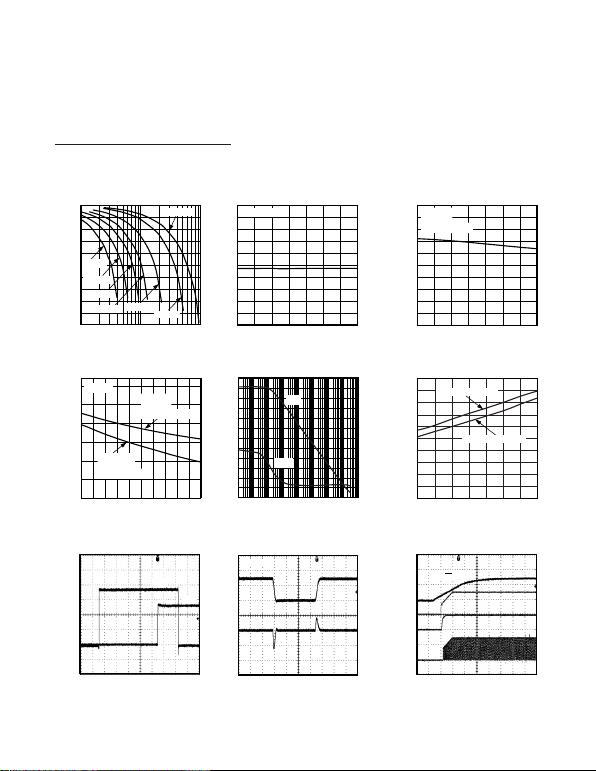OVI TO OUT DELAY THROUGH
OVERVOLTAGE COMPARATOR
MAX15004 toc16
1μs/div
VOUT
2V/div
VOVI
500mV/div
VOUT
VOVI
DRIVER OUTPUT PEAK SOURCE
AND SINK CURRENT
MAX15004 toc17
400ns/div
VOUT
5V/div
IOUT
1A/div
COUT = 10nF
POWER-UP SEQUENCE THROUGH VIN
MAX15004 toc18
2ms/div
VIN
10V/div
VCC
5V/div
REG5
5V/div
VOUT
5V/div
VON/OFF = 5V
4.5V to 40V Input Automotive
Flyback/Boost/SEPIC Power-Supply Controllers
MAX15005 MAXIMUM DUTY CYCLE
vs. OUTPUT FREQUENCY (fOUT)
OUTPUT FREQUENCY (kHz)
MAXIMUM DUTY CYCLE (%)
MAX15004 toc10
50
55
60
65
70
75
80
85
90
95
100
10 100 1000
CT = 220pF
CT = 1500pF
CT = 1000pF CT = 560pF
CT = 2200pF
CT = 3300pF
CT = 100pF
MAX15004 MAXIMUM DUTY CYCLE
vs. TEMPERATURE
TEMPERATURE (°C)
MAXIMUM DUTY CYCLE (%)
MAX15004 toc11
-40 -15 10 35 60 85 110 135
45
46
47
48
49
50
51
52
53
54
55
fOUT = 75kHz
MAX15005 MAXIMUM DUTY CYCLE
vs. TEMPERATURE
TEMPERATURE (°C)
MAXIMUM DUTY CYCLE (%)
MAX15004 toc12
-40 -15 10 35 60 85 110 135
65
67
69
71
73
75
77
79
81
83
85
CT = 560pF
RT = 13.7kΩ
fOSC = fOUT = 150kHz
MAXIMUM DUTY CYCLE
vs. fSYNC/fOSC RATIO
fSYNC/fOSC RATIO
MAXIMUM DUTY CYCLE (%)
MAX15004 toc13
1.0 1.1 1.2 1.3 1.4 1.5 1.6 1.7 1.8 1.9 2.0
50
55
60
65
70
75
80
CRTCT = 220pF
RRTCT = 10kΩ
fOSC = fOUT = 418kHz
CT = 560pF
RT = 10kΩ
fOSC = fOUT = 180kHz
MAX15005
ERROR AMPLIFIER OPEN-LOOP GAIN
AND PHASE vs. FREQUENCY
FREQUENCY (Hz)
GAIN (dB)
MAX15004 toc14
PHASE (DEGREES)
60
100
140
180
220
260
300
340
-10
0
10
20
30
40
50
60
70
80
90
100
110
0.1 1 10 100 1k 10k 100k 1M 10M
GAIN
PHASE
CS-TO-OUT DELAY vs. TEMPERATURE
TEMPERATURE (°C)
CS-TO-OUT DELAY (ns)
MAX15004 toc15
10
0
20
30
40
50
60
70
80
90
100
-40 -15 10 35 60 85 110 135
VCS OVERDRIVE = 190mV
VCS OVERDRIVE = 50mV
Typical Operating Characteristics (continued)
(VIN = 14V, CIN = 0.1μF, CVCC = 0.1μF // 1μF, CREG5 = 1μF, VON/OFF = 5V, CSS = 0.01μF, CSLOPE = 100pF, RT = 13.7kΩ,
CT = 560pF. TA = +25°C, unless otherwise noted.)
MAX15004A/B/MAX15005A/B
Maxim Integrated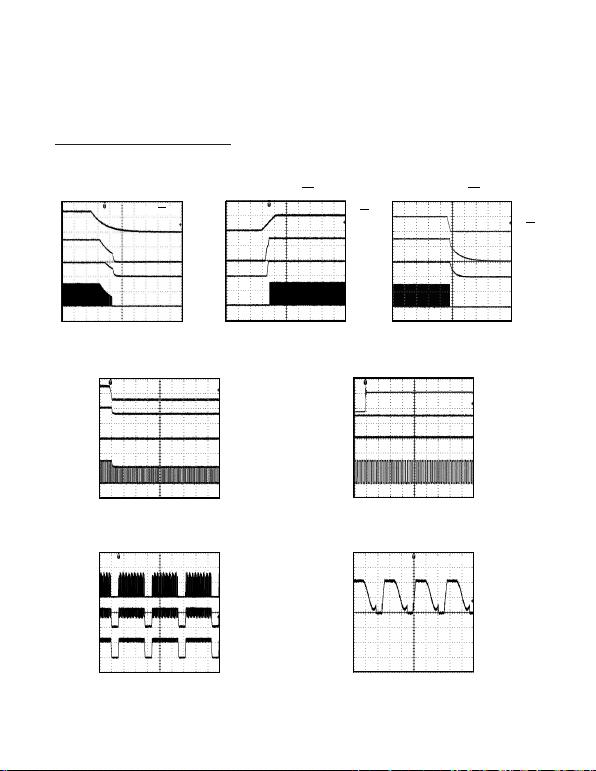4.5V to 40V Input Automotive
Flyback/Boost/SEPIC Power-Supply Controllers
POWER-DOWN SEQUENCE THROUGH VIN
MAX15004 toc19
4ms/div
VIN
10V/div
VCC
5V/div
REG5
5V/div
VOUT
5V/div
VON/OFF = 5V
POWER-UP SEQUENCE
THROUGH ON/OFF
MAX15004 toc20
1ms/div
ON/OFF
5V/div
VCC
5V/div
REG5
5V/div
VOUT
5V/div
POWER-DOWN SEQUENCE
THROUGH ON/OFF
MAX15004 toc21
400ms/div
ON/OFF
5V/div
VCC
5V/div
REG5
5V/div
VOUT
5V/div
Typical Operating Characteristics (continued)
(VIN = 14V, CIN = 0.1μF, CVCC = 0.1μF // 1μF, CREG5 = 1μF, VON/OFF = 5V, CSS = 0.01μF, CSLOPE = 100pF, RT = 13.7kΩ,
CT = 560pF. TA = +25°C, unless otherwise noted.)
HICCUP MODE FOR FLYBACK CIRCUIT
(FIGURE 7)
MAX15004 toc24
10ms/div
VCS
200mV/div
VANODE
1V/div
ISHORT
500mA/div
DRAIN WAVEFORM IN
FLYBACK CONVERTER (FIGURE 7)
MAX15004 toc25
4μs/div
10V/div
LINE TRANSIENT FOR VIN STEP
FROM 14V TO 5.5V
MAX15004 toc22
100μs/div
VIN
10V/div
VCC
5V/div
REG5
5V/div
VOUT
5V/div
LINE TRANSIENT FOR VIN STEP
FROM 14V TO 40V
MAX15004 toc23
100μs/div
VIN
20V/div
VCC
5V/div
REG5
5V/div
VOUT
5V/div
MAX15004A/B/MAX15005A/B
8
Maxim Integrated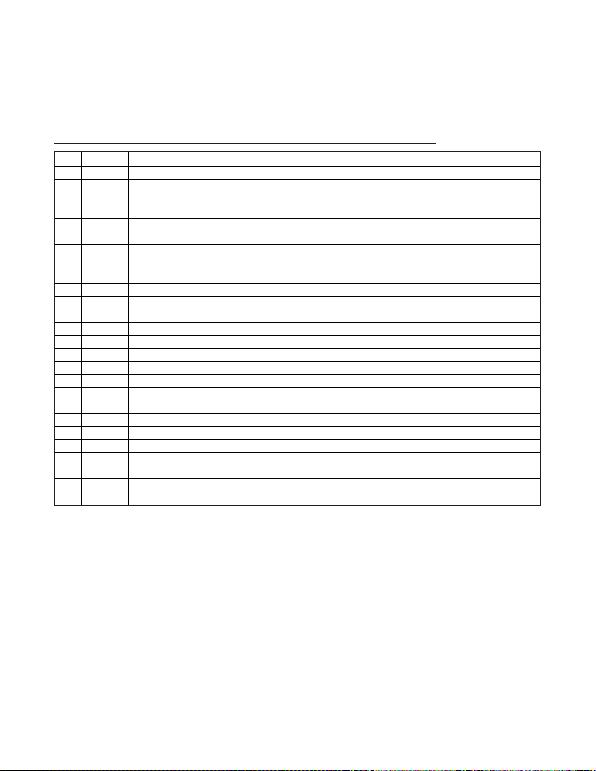4.5V to 40V Input Automotive
Flyback/Boost/SEPIC Power-Supply Controllers
Pin Description
PIN NAME FUNCTION
1 IN Input Power Supply. Bypass IN with a minimum 0.1μF ceramic capacitor to PGND.
2 ON/OFF
ON/OFF Input. Connect ON/OFF to IN for always-on operation. To externally program the UVLO threshold of the
IN supply, connect a resistive divider between IN, ON/OFF, and SGND. Pull ON/OFF to SGND to disable the
controller.
3 OVI Overvoltage Comparator Input. Connect a resistive divider between the output of the power supply, OVI, and
SGND to set the output overvoltage threshold.
4 SLOPE
Programmable Slope Compensation Capacitor Input. Connect a capacitor (CSLOPE) to SGND to set the amount
of slope compensation.
Slope compensation = (2.5 x 10-9) / CSLOPE mV/μs with CSLOPE in farads.
5 N.C. No Connection. Not internally connected.
6 RTCT Oscillator-Timing Network Input. Connect a resistor from RTCT to REG5 and a capacitor from RTCT to SGND to
set the oscillator frequency (see the Oscillator Frequency/External Synchronization section).
7 SGND Signal Ground. Connect SGND to SGND plane.
8 SYNC External-Clock Synchronization Input. Connect SYNC to SGND when not using an external clock.
9 SS Soft-Start Capacitor Input. Connect a capacitor from SS to SGND to set the soft-start time interval.
10 FB Internal Error-Amplifier Inverting Input. The noninverting input is internally connected to SS.
11 COMP Error-Amplifier Output. Connect the frequency compensation network between FB and COMP.
12 CS Current-Sense Input. The current-sense signal is compared to a signal proportional to the error-amplifier output
voltage.
13 REG5 5V Low-Dropout Regulator Output. Bypass REG5 with a 1μF ceramic capacitor to SGND.
14 PGND Power Ground. Connect PGND to the power ground plane.
15 OUT Gate Driver Output. Connect OUT to the gate of the external n-channel MOSFET.
16 VCC 7.4V Low-Dropout Regulator Output—Driver Power Source. Bypass VCC with 0.1μF and 1μF or higher ceramic
capacitors to PGND.
—EP
Exposed Pad (MAX15004A/MAX15005A only). Connect EP to the SGND plane to improve thermal performance.
Do not use the EP as an electrical connection.
MAX15004A/B/MAX15005A/B
Maxim Integrated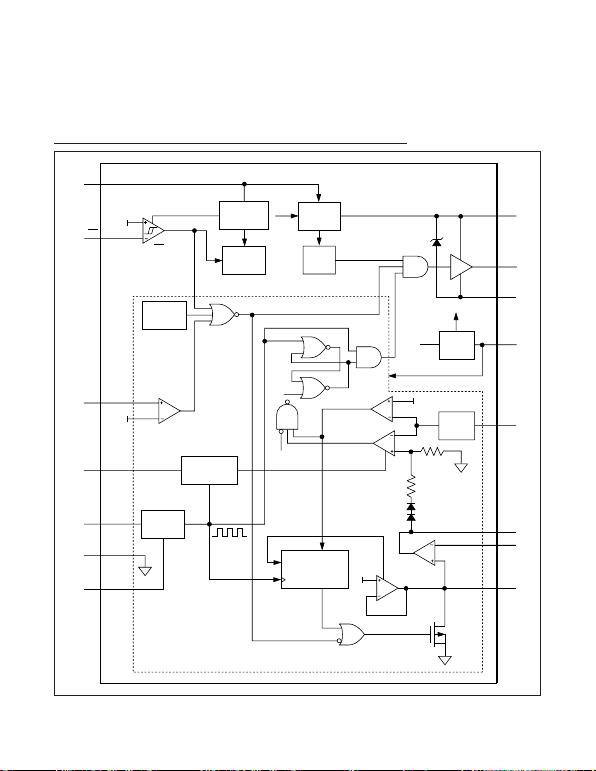4.5V to 40V Input Automotive
Flyback/Boost/SEPIC Power-Supply Controllers
Functional Diagram
MAX15004A/B
MAX15005A/B
PREREGULATOR
REFERENCE
SLOPE
COMPENSATION
EAMP
REF-AMP
THERMAL
SHUTDOWN
OV-COMP
ON/OFF
COMP
OSCILLATOR
7
CONSECUTIVE
EVENTS
COUNTER
7.4V LDO
REG
5V LDO
REG
UVB
VCC
UVB
10.5V
30mA
CLAMP
3.5V
UVLO
50ns
DELAY
DRIVER
R
0.3V
2R
SS_OK
RESET
1.228V
1.228V
1.228V
1
2
3
4
6
7
8
SYNC
SGND
RTCT
SLOPE
OVI
ON/OFF
IN
VCC
16
OUT
15
PGND
14
REG5
13
CS
12
COMP
11
FB
10
SS
9
OFF
OFF
SET
RESET
OVRLD
CLK
OVRLD
PWM-
COMP
ILIMIT
COMP
MAX15004A/B/MAX15005A/B
10
Maxim Integrated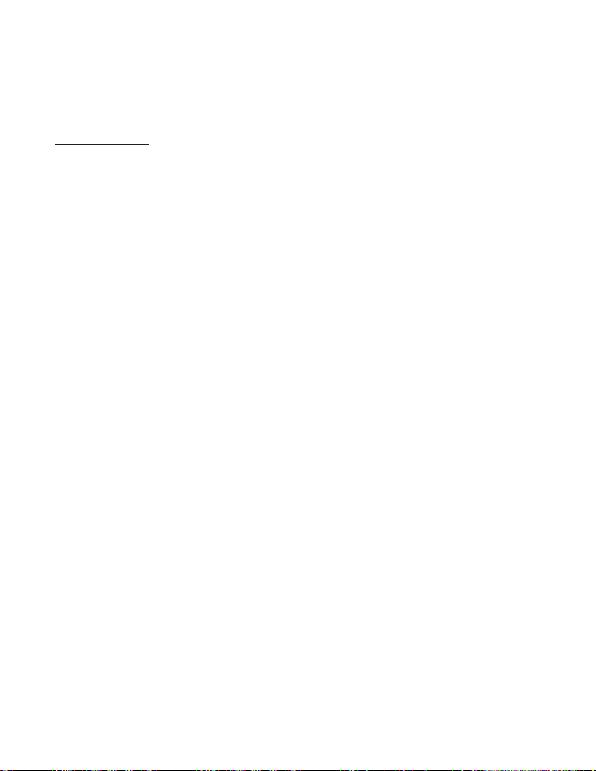4.5V to 40V Input Automotive
Flyback/Boost/SEPIC Power-Supply Controllers
Detailed Description
The MAX15004A/B/MAX15005A/B are high-perfor-
mance, current-mode PWM controllers for wide input-
voltage range isolated/nonisolated power supplies.
These controllers are for use as general-purpose boost,
flyback, and SEPIC controllers. The input voltage range
of 4.5V to 40V makes it ideal in automotive applications
such as vacuum fluorescent display (VFD) power sup-
plies. The internal low-dropout regulator (VCC regula-
tor) enables the MAX15004A/B/MAX15005A/B to
operate directly from an automotive battery input. The
input operating range can be as low as 2.5V when an
external source (e.g. bootstrap winding output) is
applied at the VCC input. The 2.5V to 40V input voltage
range allows device operation from cold crank to auto-
The undervoltage lockout (ON/OFF) allows the devices
to program the input-supply startup voltage and ensures
predictable operation during brownout conditions.
The devices contain two internal regulators, VCC and
REG5. The VCC regulator output voltage is set at 7.4V
and REG5 regulator output voltage at 5V ±2%. The
VCC output includes a 10.4V clamp that is capable of
sinking up to 30mA current. The input undervoltage
lockout (UVLO) circuit monitors the VCC voltage and
turns off the converter when the VCC voltage drops
below 3.5V (typ). See the
Internal Regulators V
CC
and
REG5
section for a method to obtain lower than 4.5V
input operation with the MAX15004/MAX15005.
An external resistor and capacitor network programs
the switching frequency from 15kHz to 500kHz. The
MAX15004A/B/MAX15005A/B provide a SYNC input for
synchronization to an external clock. The OUT (FET-dri-
ver output) duty cycle for the MAX15004A/B is 50%.
The maximum duty cycle can be set on MAX15005A/B
by selecting the right combination of RT and CT. The
RTCT discharge current is trimmed to 2%, allowing
accurate setting of the duty cycle for the MAX15005.
An internal slope-compensation circuit stabilizes the
current loop when operating at higher duty cycles and
can be programmed externally.
The MAX15004/MAX15005 include an internal error
amplifier with 1% accurate reference to regulate the
output in nonisolated topologies using a resistive
divider. The internal reference connected to the nonin-
verting input of the error amplifier can be increased in a
controlled manner to obtain soft-start. A capacitor con-
nected at SS to ground programs soft-start to reduce
inrush current and prevent output overshoot.
The MAX15004/MAX15005 include protection features
like hiccup current limit, output overvoltage, and thermal
shutdown. The hiccup current-limit circuit reduces the
MAX15004/MAX15005 converter during severe fault con-
ditions. The overvoltage circuit senses the output using
the path different from the feedback path to provide
meaningful overvoltage protection. During continuous
high input operation, the power dissipation into the
MAX15004/MAX15005 could exceed its limit. Internal
thermal shutdown protection safely turns off the converter
when the junction heats up to 160°C.
Current-Mode Control Loop
The advantages of current-mode control overvoltage-
mode control are twofold. First, there is the feed-for-
ward characteristic brought on by the controller’s ability
to adjust for variations in the input voltage on a cycle-
by-cycle basis. Secondly, the stability requirements of
the current-mode controller are reduced to that of a sin-
gle-pole system unlike the double pole in voltage-mode
control.
The MAX15004/MAX15005 offer peak current-mode
control operation to make the power supply easy to
design with. The inherent feed-forward characteristic is
useful especially in an automotive application where the
input voltage changes fast during cold-crank and load
dump conditions. While the current-mode architecture
offers many advantages, there are some shortcomings.
For higher duty-cycle and continuous conduction mode
operation where the transformer does not discharge
during the off duty cycle, subharmonic oscillations
appear. The MAX15004/MAX15005 offer programmable
slope compensation using a single capacitor. Another
issue is noise due to turn-on of the primary switch that
may cause the premature end of the on cycle. The cur-
rent-limit and PWM comparator inputs have leading-
edge blanking. All the shortcomings of the
current-mode control are addressed in the MAX15004/
MAX15005, making it ideal to design for automotive
power conversion applications.
Internal Regulators VCC and REG5
The internal LDO converts the automotive battery volt-
age input to a 7.4V output voltage (VCC). The VCC out-
put is set at 7.4V and operates in a dropout mode at
input voltages below 7.5V. The internal LDO is capable
of delivering 20mA current, enough to provide power to
internal control circuitry and the gate drive. The regulat-
ed VCC keeps the driver output voltage well below the
absolute maximum gate voltage rating of the MOSFET
especially during the double battery and load dump
conditions. An auxiliary winding output can be fed to
the VCC output once the power supply is turned on.
The bootstrap winding is not necessary for proper
MAX15004A/B/MAX15005A/B
11
Maxim Integrated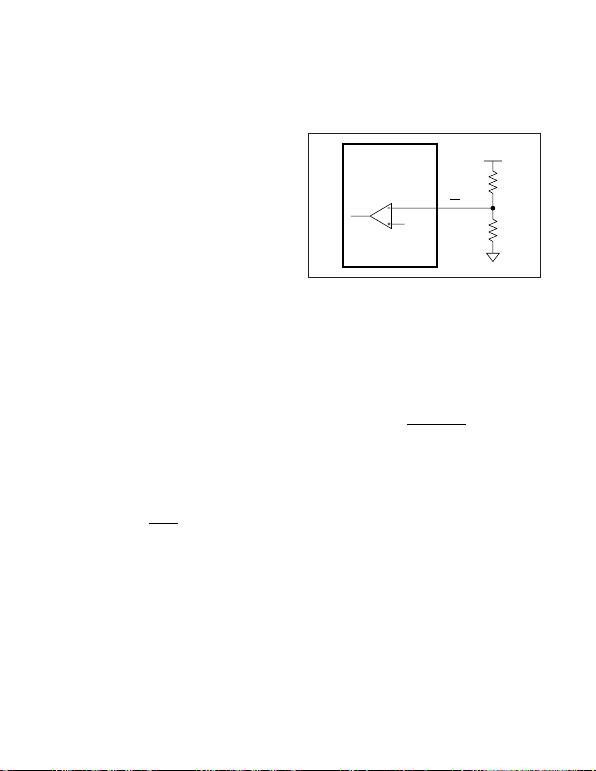4.5V to 40V Input Automotive
Flyback/Boost/SEPIC Power-Supply Controllers
operation of the power supply; however, to reduce the
power dissipation of the internal LDO, it can be dis-
abled by applying an external voltage higher than 7.4V
at VCC (LDO output). The LDO then stops drawing cur-
rent from IN, thereby reducing the power dissipation in
the IC. The VCC voltage is clamped to 10.4V with 30mA
current sink in case there is a higher voltage at the bias
winding. This feature is useful in applications with con-
tinuous higher input voltage.
The second 5V LDO regulator from VCC to REG5 pro-
vides power to the internal control circuits. This LDO can
also be used to source 15mA of external load current.
Bypass VCC and REG5 with a parallel combination of
1μF and 0.1μF low-ESR ceramic capacitors. Additional
capacitors (up to 22μF) at VCC can be used although
they are not necessary for proper operation of the
MAX15004/MAX15005.
Startup Operation/UVLO/ON/
OFF
The MAX15004A/B/MAX15005A/B feature two under-
voltage lockouts (UVLO). The internal UVLO monitors
the VCC-regulator and turns on the converter once VCC
rises above 3.5V. The internal UVLO circuit has about
0.5V hysteresis to avoid chattering during turn-on. Once
the power is on and the bootstrapped voltage feeds
VCC, IN voltage can drop below 4V. This feature pro-
vides operation at a cold-crank voltage as low as 2.5V.
An external undervoltage lockout can be achieved by
controlling the voltage at the ON/OFF input. The
ON/OFF input threshold is set at 1.23V (rising) with
75mV hysteresis.
Before any operation can commence, the ON/OFF volt-
age must exceed the 1.23V threshold.
Calculate R1 in Figure 1 by using the following formula:
where VUVLO is the ON/OFF’s 1.23V rising threshold,
and VON is the desired input startup voltage. Choose
an R2 value in the 100kΩrange. The UVLO circuits
keep the PWM comparator, ILIM comparator, oscillator,
and output driver shut down to reduce current con-
sumption (see the
Functional Diagram
). The ON/OFF
input can be used to disable the MAX15004/MAX15005
and reduce the standby current to less than 20μA.
Soft-Start
The MAX15004/MAX15005 are provided with an exter-
nally adjustable soft-start function, saving a number of
external components. The SS is a 1.228V reference
bypass connection for the MAX15004A/B/MAX15005A/B
and also controls the soft-start period. At startup, after
VIN is applied and the UVLO thresholds are reached,
the device enters soft-start. During soft-start, 15μA is
sourced into the capacitor (CSS) connected from SS to
GND causing the reference voltage to ramp up slowly.
The HICCUP mode of operation is disabled during soft-
start. When VSS reaches 1.228V, the output as well as
the HICCUP mode become fully active. Set the soft-start
time (tSS) using following equation:
where tSS is in seconds and CSS is in farads.
The soft-start programmability is important to control the
input inrush current issue and also to avoid the
MAX15004/MAX15005 power supply from going into the
unintentional hiccup during the startup. The required
soft-start time depends on the topology used, current-
limit setting, output capacitance, and the load condition.
Oscillator Frequency/
External Synchronization
Use an external resistor and capacitor at RTCT to pro-
gram the MAX15004A/B/MAX15005A/B internal oscillator
frequency from 15kHz to 1MHz. The MAX15004A/B out-
put switching frequency is one-half the programmed
oscillator frequency with a 50% maximum duty-cycle
limit. The MAX15005A/B output switching frequency is
the same as the oscillator frequency. The RC network
connected to RTCT controls both the oscillator frequency
and the maximum duty cycle. The CT capacitor charges
and discharges from (0.1 x VREG5) to (0.55 x VREG5). It
charges through RT and discharges through an internal
trimmed controlled current sink. The maximum duty
cycle is inversely proportional to the discharge time
tVC
A
SS SS
=×
×
()
123
15 10 6
.()
RV
VR
ON
UVLO
112=−
×
Figure 1. Setting the MAX15004A/B/MAX15005A/B
Undervoltage Lockout Threshold
MAX15004A/B
MAX15005A/B
ON/OFF
R1
1.23V R2
VIN
MAX15004A/B/MAX15005A/B
12
Maxim Integrated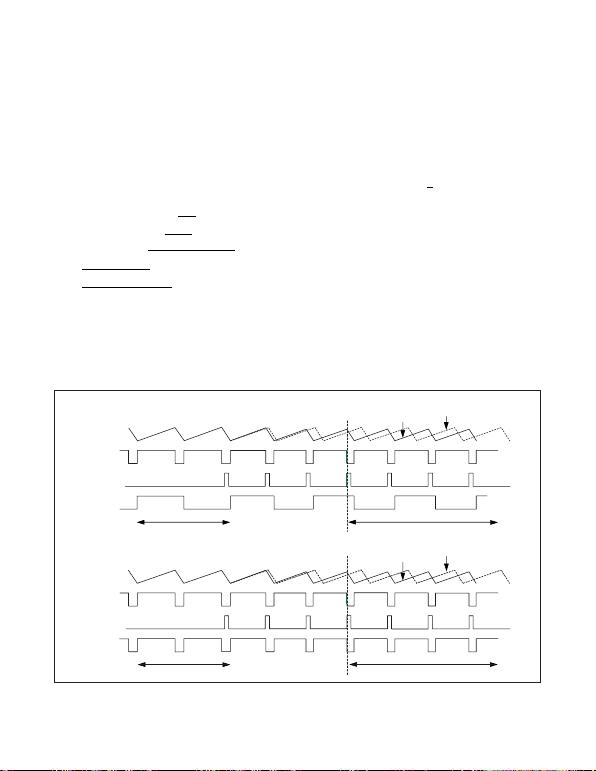4.5V to 40V Input Automotive
Flyback/Boost/SEPIC Power-Supply Controllers
(tDISCHARGE). See Figures 3a and 3b for a coarse selec-
tion of capacitor values for a given switching frequency
and maximum duty cycle and then use the following
equations to calculate the resistor value to fine-tune the
switching frequency and verify the worst-case maximum
duty cycle.
where fOSC is the oscillator frequency, RT is a resistor
connected from RTCT to REG5, and CT is a capacitor
connected from RTCT to SGND. Verify that the oscilla-
tor frequency value meets the target. Above calcula-
tions could be repeated to fine-tune the switching
frequency.
The MAX15004A/B is a 50% maximum duty-cycle part,
while the MAX15005A/B is 100% maximum duty-cycle
part.
for the MAX15004A/B and
for the MAX15005A/B.
The MAX15004A/B/MAX15005A/B can be synchronized
using an external clock at the SYNC input. For proper
frequency synchronization, SYNC’s input frequency must
be at least 102% of the programmed internal oscillator
frequency. Connect SYNC to SGND when not using an
external clock. A rising clock edge on SYNC is interpret-
ed as a synchronization input. If the SYNC signal is lost,
the internal oscillator takes control of the switching rate,
returning the switching frequency to that set by RC net-
work connected to RTCT. This maintains output regula-
tion even with intermittent SYNC signals.
ff
OUT OSC
=
ff
OUT OSC
=1
2
tD
f
RT t
CT
t
CHARGE MAX
OSC
CHARGE
DISCHARGE
=
=×
=
07.
2225
1 33 10 3 375
3
.()
(. ( ) ) . ( )
VRTCT
ART V
fOS
××
××
CC CHARGE DISCHARGE
tt Us
=+
1................... ee This Equation If f kHz
tt
OSC
CHARGE DISCHA
+
500
1
RRGE OSC
ns Use This Equation If f k
+>
160 500....... HHz
Figure 2. Timing Diagram for Internal Oscillator vs. External SYNC and DMAX Behavior
RTCT
CLKINT
SYNC
OUT
RTCT
CLKINT
SYNC
OUT
D = 50% D = 50%
D = 81.25% D = 80%
WITH SYNC
INPUT
MAX15004A/B (DMAX = 50%)
MAX15005A/B (DMAX = 81%)
WITHOUT
SYNC INPUT
WITH SYNC
INPUT
WITHOUT
SYNC INPUT
MAX15004A/B/MAX15005A/B
13
Maxim Integrated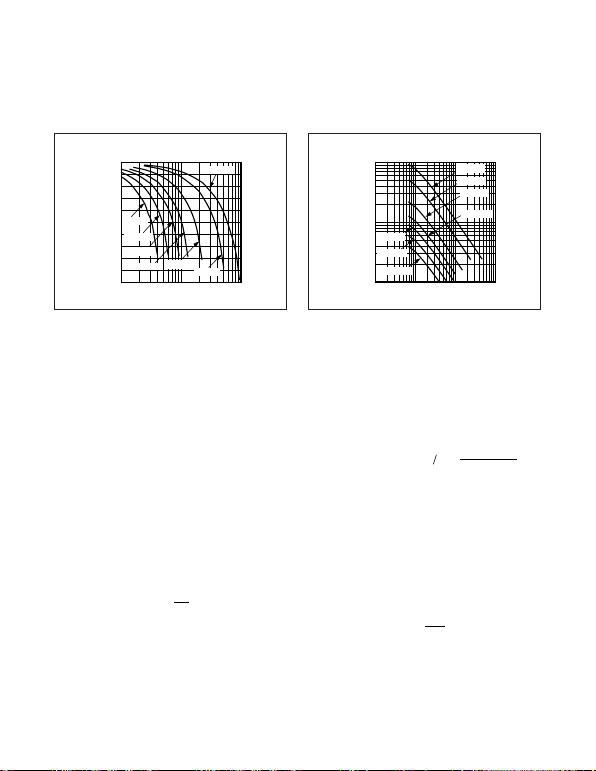4.5V to 40V Input Automotive
Flyback/Boost/SEPIC Power-Supply Controllers
n-Channel MOSFET Driver
OUT drives the gate of an external n-channel MOSFET.
internally set to approximately 7.4V. If an external voltage
higher than 7.4V is applied at VCC (up to 10V), it appears
as the peak gate drive voltage. The regulated VCC volt-
age keeps the OUT voltage below the maximum gate
voltage rating of the external MOSFET. OUT can source
750mA and sink 1000mA peak current. The average cur-
rent sourced by OUT depends on the switching frequen-
cy and total gate charge of the external MOSFET.
Error Amplifier
The MAX15004A/B/MAX15005A/B include an internal
error amplifier. The noninverting input of the error
amplifier is connected to the internal 1.228V reference
and feedback is provided at the inverting input. High
100dB open-loop gain and 1.6MHz unity-gain band-
width allow good closed-loop bandwidth and transient
response. Moreover, the source and sink current capa-
bility of 2mA provides fast error correction during the
output load transient. For Figure 5, calculate the power-
supply output voltage using the following equation:
where VREF = 1.228V. The amplifier’s noninverting input
is internally connected to a soft-start circuit that gradu-
ally increases the reference voltage during startup. This
forces the output voltage to come up in an orderly and
well-defined manner under all load conditions.
Slope Compensation
The MAX15004A/B/MAX15005A/B use an internal ramp
generator for slope compensation. The internal ramp
signal resets at the beginning of each cycle and slews
at the rate programmed by the external capacitor con-
nected to SLOPE. The amount of slope compensation
needed depends on the downslope of the current
slew rate up to 110mV/μs using the following equation:
where CSLOPE is the external capacitor at SLOPE in
Current Limit
The current-sense resistor (RCS), connected between
the source of the MOSFET and ground, sets the current
limit. The CS input has a voltage trip level (VCS) of
305mV. The current-sense threshold has 5% accuracy.
Set the current-limit threshold 20% higher than the peak
switch current at the rated output power and minimum
input voltage. Use the following equation to calculate
the value of RS:
where IPRI is the peak current that flows through the
MOSFET at full load and minimum VIN.
RV
I
SCS
PRI
=
Slope compensation mV s A
CSLOPE
()
.()
μ= ×
25 10 9
VR
RV
OUT A
BREF
=+
1
Figure 3a. MAX15005 Maximum Duty Cycle vs. Output
Frequency.
MAX15005 MAXIMUM DUTY CYCLE
vs. OUTPUT FREQUENCY (fOUT)
OUTPUT FREQUENCY (kHz)
MAXIMUM DUTY CYCLE (%)
50
55
60
65
70
75
80
85
90
95
100
10 100 1000
CT = 220pF
CT = 1500pF
CT = 1000pF CT = 560pF
CT = 2200pF
CT = 3300pF
CT = 100pF
Figure 3b. Oscillator Frequency vs. RT/CT
OSCILLATOR FREQUENCY (fOSC)
vs. RT/CT
RT (kΩ)
OSCILLATOR FREQUENCY (kHz)
1 10 100 1000
10
100
1000
CT = 220pF
CT = 1500pF
CT = 1000pF
CT = 560pF
CT = 2200pF
CT = 3300pF
CT = 100pF
MAX15004A/B/MAX15005A/B
14
Maxim Integrated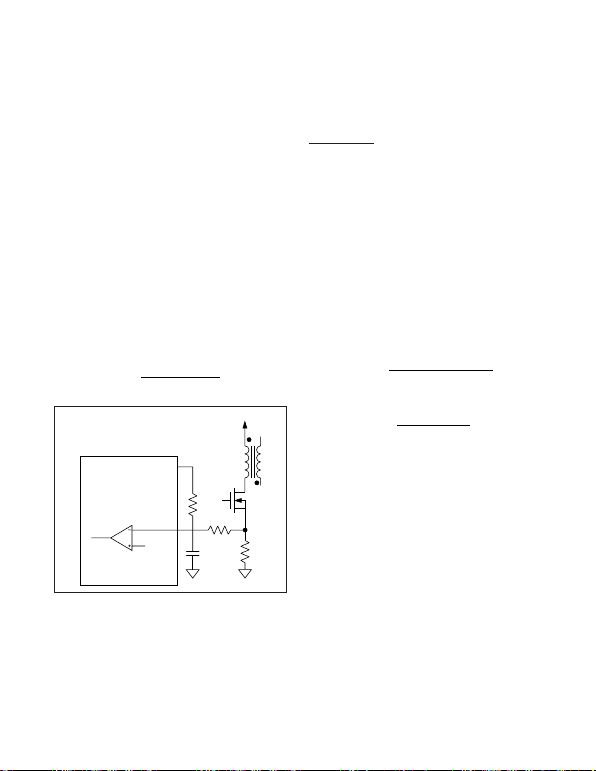4.5V to 40V Input Automotive
Flyback/Boost/SEPIC Power-Supply Controllers
When the voltage produced by this current (through the
current-sense resistor) exceeds the current-limit com-
parator threshold, the MOSFET driver (OUT) quickly ter-
minates the on-cycle. In most cases, a short-time
constant RC filter is required to filter out the leading-
edge spike on the sense waveform. The amplitude and
width of the leading edge depends on the gate capaci-
tance, drain capacitance (including interwinding capac-
itance), and switching speed (MOSFET turn-on time).
Set the RC time constant just long enough to suppress
ing spike at the highest input and rated output load to
determine the value of the RC filter.
The low 305mV current-limit threshold reduces the
power dissipation in the current-sense resistor. The cur-
rent-limit threshold can be further reduced by adding
an offset to the CS input from REG5 voltage. Do not
reduce the current-limit threshold below 150mV as it
may cause noise issues. See Figure 4. For a new value
of the current-limit threshold (VCS-LOW), calculate the
value of R1 using the following equation.
Applications Information
Boost Converter
The MAX15004A/B/MAX15005A/B can be configured
for step-up conversion. The boost converter output can
be fed back to VCC (see Figure 5) so that the controller
can function even during cold-crank input voltage
(2.5V). Use a Schottky diode (DVIN) in the VIN path to
avoid backfeeding the input source. A current-limiting
resistor (RVCC) is also needed from the boost converter
output to VCC depending upon the boost converter out-
put voltage. The total current sink into VCC must be lim-
ited to 30mA. Use the equations in the following
sections to calculate RVCC, inductor (LMIN), input
capacitor (CIN), and output capacitor (COUT) when
using the converter in boost operation.
Inductor Selection in Boost Configuration
Using the following equation, calculate the minimum
inductor value so that the converter remains in continu-
ous mode operation at minimum output current (IOMIN).
where:
and
The higher value of IOMIN reduces the required induc-
tance; however, it increases the peak and RMS currents
in the switching MOSFET and inductor. Use IOMIN from
10% to 25% of the full load current. The VDis the for-
ward voltage drop of the external Schottky diode, D is
the duty cycle, and VDS is the voltage drop across the
external switch. Select the inductor with low DC resis-
tance and with a saturation current (ISAT) rating higher
than the peak switch current limit of the converter.
I (0.1 I ) to (0.25 I
OMIN O O
×)
DVVV
OUT D IN
=+
VVVV
OUT D DS
+
LVD
2f V I
MIN IN
OUT OUT OMIN
=××
×× ×
2η
RR
V Low
CS
CS
1475
0 290
=×
.
.
Figure 4. Reducing Current-Sense Threshold
MAX15004A/B
MAX15005A/B
REG5
0.3V
CURRENT-LIMIT
COMPARATOR
RS
RCS
CCS
VIN
N
R1
MAX15004A/B/MAX15005A/B
Maxim Integrated
15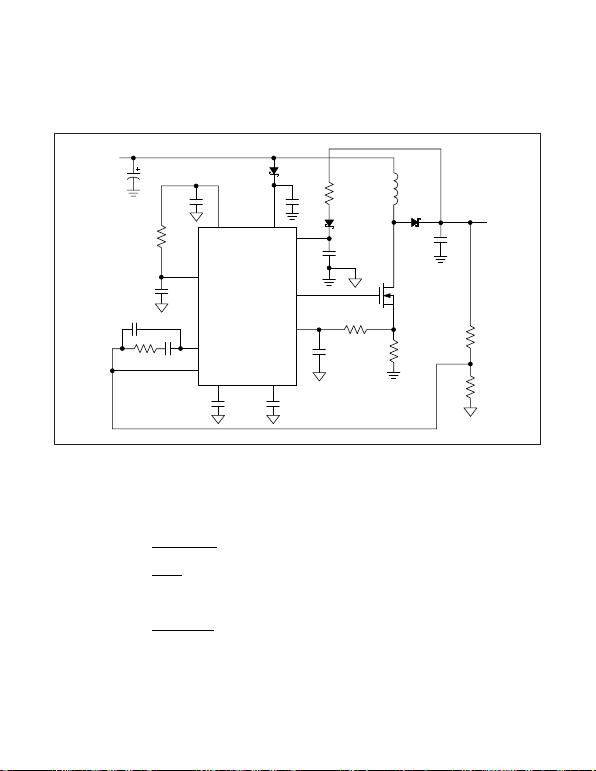Input Capacitor Selection in Boost Configuration
The input current for the boost converter is continuous
and the RMS ripple current at the input capacitor is low.
Calculate the minimum input capacitor value and maxi-
mum ESR using the following equations:
where:
VDS is the total voltage drop across the external MOS-
FET plus the voltage drop across the inductor ESR. ΔIL
is peak-to-peak inductor ripple current as calculated
above. ΔVQis the portion of input ripple due to the
capacitor discharge and ΔVESR is the contribution due
to ESR of the capacitor. Assume the input capacitor rip-
ple contribution due to ESR (ΔVESR) and capacitor dis-
charge (ΔVQ) is equal when using a combination of
ceramic and aluminum capacitors. During the convert-
er turn-on, a large current is drawn from the input
source especially at high output to input differential.
The MAX15004/MAX15005 are provided with a pro-
grammable soft-start, however, a large storage capaci-
tor at the input may be necessary to avoid chattering
due to finite hysteresis.
Output Capacitor Selection in Boost Configuration
For the boost converter, the output capacitor supplies
the load current when the main switch is on. The
required output capacitance is high, especially at higher
duty cycles. Also, the output capacitor ESR needs to be
low enough to minimize the voltage drop due to the ESR
while supporting the load current. Use the following
equations to calculate the output capacitor, for a speci-
fied output ripple. All ripple values are peak-to-peak.
I(V V
LIN DS
=
Δ))D
Lf
OUT
×
×
CID
4f V
ESR V
I
IN L
OUT Q
ESR
L
=×
××
=
Δ
Δ
Δ
Δ
4.5V to 40V Input Automotive
Flyback/Boost/SEPIC Power-Supply Controllers
Figure 5. Application Schematic
MAX15004A/B
MAX15005A/B
IN
CVCC
4.7μF
RVCC
DVCC
DVIN
RCS
D3
PGND
Q
L
RS
COUT
RB
RA
CVIN
1μF
REG5
SS
SLOPE
11
6
13
16
15
12
1
49
10
RT
CT
VCC
OUT
CS
VOUT
RTCT
COMP
CREG5
0.1μF
VIN
18V
CIN
CCS
CSS
CSLOPE
RF CF
FB
CFF
MAX15004A/B/MAX15005A/B
16
Maxim Integrated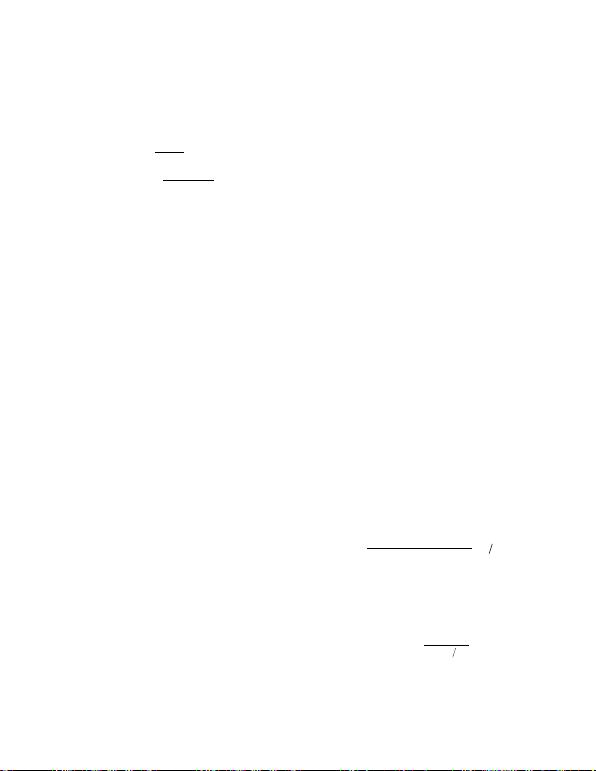IOis the load current, ΔVQis the portion of the ripple due
to the capacitor discharge, and ΔVESR is the contribution
due to the ESR of the capacitor. DMAX is the maximum
duty cycle at the minimum input voltage. Use a combina-
tion of low-ESR ceramic and high-value, low-cost alu-
minum capacitors for lower output ripple and noise.
Calculating Power Loss in Boost Converter
The MAX15004A/MAX15005A devices are available in
a thermally enhanced package and can dissipate up to
1.7W at +70°C ambient temperature. The total power
dissipation in the package must be limited so that the
junction temperature does not exceed its absolute max-
imum rating of +150°C at maximum ambient tempera-
ture; however, Maxim recommends operating the
junction at about +125°C for better reliability.
The average supply current (IDRIVE-GATE) required by
the switch driver is:
where Qgis total gate charge at 7.4V, a number avail-
able from MOSFET datasheet.
The supply current in the MAX15004A/B/MAX15005A/B
is dependent on the switching frequency. See the
Typical Operating Characteristics
to find the supply
current ISUPPLY of the MAX15004A/B/MAX15005A/B at
a given operating frequency. The total power dissipa-
tion (PT) in the device due to supply current (ISUPPLY)
and the current required to drive the switch (IDRIVE-
GATE) is calculated using following equation.
MOSFET Selection in Boost Converter
The MAX15004A/B/MAX15005A/B drive a wide variety of
n-channel power MOSFETs. Since VCC limits the OUT
output peak gate-drive voltage to no more than 11V, a
12V (max) gate voltage-rated MOSFET can be used with-
out an additional clamp. Best performance, especially at
low-input voltages (5VIN), is achieved with low-threshold
n-channel MOSFETs that specify on-resistance with a
gate-source voltage (VGS) of 2.5V or less. When selecting
the MOSFET, key parameters can include:
1) Total gate charge (Qg).
2) Reverse-transfer capacitance or charge (CRSS).
3) On-resistance (RDS(ON)).
4) Maximum drain-to-source voltage (VDS(MAX)).
5) Maximum gate frequencies threshold voltage
(VTH(MAX)).
At high switching, dynamic characteristics (parameters 1
and 2 of the above list) that predict switching losses
have more impact on efficiency than RDS(ON), which pre-
dicts DC losses. Qgincludes all capacitances associat-
ed with charging the gate. The VDS(MAX) of the selected
MOSFET must be greater than the maximum output volt-
age setting plus a diode drop. The 10V additional margin
is recommended for spikes at the MOSFET drain due to
the inductance in the rectifier diode and output capacitor
path. In addition, Qghelps predict the current needed to
drive the gate at the selected operating frequency when
the internal LDO is driving the MOSFET.
Slope Compensation in Boost Configuration
The MAX15004A/B/MAX15005A/B use an internal ramp
generator for slope compensation to stabilize the current
loop when operating at duty cycles above 50%. It is
than 50% duty cycle to improve the noise immunity. The
slope compensations should be optimized because too
much slope compensation can turn the converter into the
voltage-mode control. The amount of slope compensation
required depends on the downslope of the inductor cur-
rent when the main switch is off. The inductor downslope
depends on the input to output voltage differential of the
boost converter, inductor value, and the switching fre-
quency. Theoretically, the compensation slope should be
equal to 50% of the inductor downslope; however, a little
higher than 50% slope is advised.
Use the following equation to calculate the required
compensating slope (mc) for the boost converter:
The internal ramp signal resets at the beginning of
each cycle and slews at the rate programmed by the
external capacitor connected to SLOPE. Adjust the
MAX15004A/B/MAX15005A/B slew rate up to 110mV/μs
using the following equation:
where CSLOPE is the external capacitor at SLOPE in
Cmc mV s
SLOPE =×
25 10 9
.
()μ
mc VVR
LmV s
OUT IN S
=××
()
()10
2
3μ
PV I
INMAX SUPPLYTDRIVEGATE
I +
()
IQf
DRIVE GATE g OUT
ESR V
I
CID
Vf
ESR
O
OUT OMAX
QOUT
=
=×
×
Δ
Δ
4.5V to 40V Input Automotive
Flyback/Boost/SEPIC Power-Supply Controllers
MAX15004A/B/MAX15005A/B
Maxim Integrated
17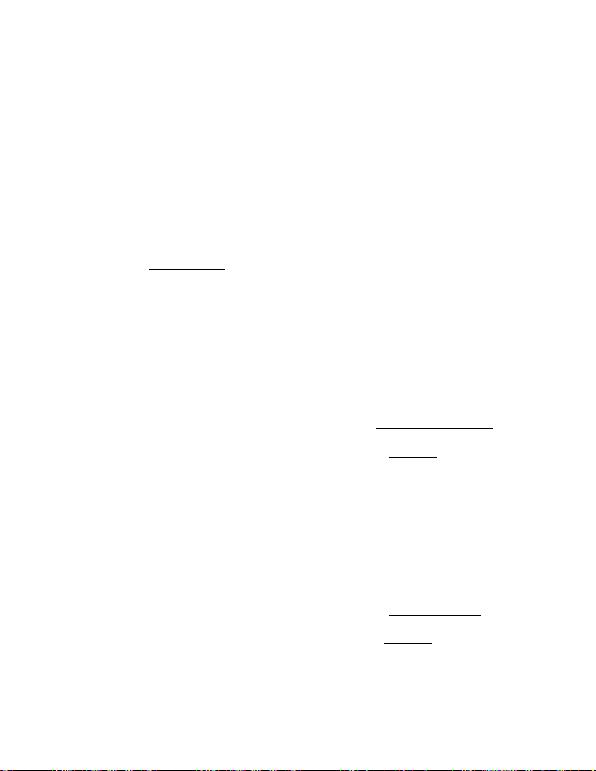4.5V to 40V Input Automotive
Flyback/Boost/SEPIC Power-Supply Controllers
Selecting V
CC
Resistor (R
VCC
)
The VCC external supply series resistor should be sized
to provide enough average current from VOUT to drive
the external MOSFET (IDRIVE) and ISUPPLY. The VCC is
clamped internally to 10.4V and capable of sinking
30mA current. The VCC resistor must be high enough to
limit the VCC sink current below 30mA at the highest
output voltage. Maintain the VCC voltage to 8V while
feeding the power from VOUT to VCC. For a regulated
output voltage of VOUT, calculate the RVCC using the
following equation:
See Figure 5 and the
Power Dissipation
section for the
values of ISUPPLY and IDRIVE.
Flyback Converter
The choice of the conversion topology is the first stage
in power-supply design. The topology selection criteria
include input voltage range, output voltage, peak cur-
rents in the primary and secondary circuits, efficiency,
form factor, and cost.
For an output power of less than 50W and a 1:2 input
voltage range with small form factor requirements, the
flyback topology is the best choice. It uses a minimum
of components, thereby reducing cost and form factor.
The flyback converter can be designed to operate
either in continuous or discontinuous mode of opera-
tion. In discontinuous mode of operation, the trans-
former core completes its energy transfer during the
off-cycle, while in continuous mode of operation, the
next cycle begins before the energy transfer is com-
plete. The discontinuous mode of operation is chosen
for the present example for the following reasons:
It maximizes the energy storage in the magnetic
component, thereby reducing size.
Simplifies the dynamic stability compensation design
(no right-half plane zero).
Higher unity-gain bandwidth.
A major disadvantage of discontinuous mode operation
is the higher peak-to-average current ratio in the primary
and secondary circuits. Higher peak-to-average current
means higher RMS current, and therefore, higher loss
and lower efficiency. For low-power converters, the
advantages of using discontinuous mode easily surpass
the possible disadvantages. Moreover, the drive capabil-
ity of the MAX15004/MAX15005 is good enough to drive
a large switching MOSFET. With the presently available
MOSFETs, power output of up to 50W is easily achiev-
able with a discontinuous mode flyback topology using
the MAX15004/MAX15005 in automotive applications.
Transformer Design
Step-by-step transformer specification design for a dis-
continuous flyback example is explained below.
Follow the steps below for the discontinuous mode
transformer:
Step 1) Calculate the secondary winding inductance
for guaranteed core discharge within a mini-
mum off-time.
Step 2) Calculate primary winding inductance for suffi-
cient energy to support the maximum load.
Step 3) Calculate the secondary and bias winding
turns ratios.
Step 4) Calculate the RMS current in the primary and
estimate the secondary RMS current.
Step 5) Consider proper sequencing of windings and
transformer construction for low leakage.
Step 1) As discussed earlier, the core must be dis-
charged during the off-cycle for discontinuous mode
operation. The secondary inductance determines the
time required to discharge the core. Use the following
equations to calculate the secondary inductance:
where:
DOFFMIN = minimum DOFF.
VD= secondary diode forward voltage drop.
IOUT = maximum output rated current.
Step 2) The rising current in the primary builds the
energy stored in the core during on-time, which is then
released to deliver the output power during the off-time.
Primary inductance is then calculated to store enough
energy during the on-time to support the maximum out-
put power.
DMAX = Maximum D.
LVD
Pf
Dt
t
PINMIN MAX
OUT OUT MAX
ON
ON
=××
××
=+
22
2
η
()
ttOFF
LVVD
If
D
SOUT D OFFMIN
OUT OUT MAX
OFF
+
()
×
()
××
2
2()
== +
t
tt
OFF
ON OFF
RV
II
VCC OUT
SUPPLY DRIVE
=+
()
()
8
MAX15004A/B/MAX15005A/B
18
Maxim Integrated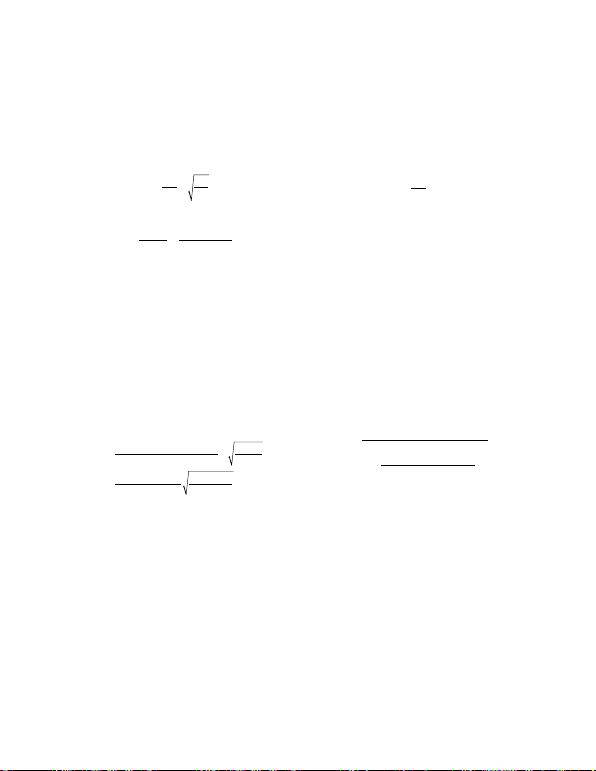4.5V to 40V Input Automotive
Flyback/Boost/SEPIC Power-Supply Controllers
Step 3) Calculate the secondary to primary turns ratio
(NSP) and the bias winding to primary turns ratio (NBP)
using the following equations:
and
The forward bias drops of the secondary diode and the
bias rectifier diode are assumed to be 0.35V and 0.7V,
respectively. Refer to the diode manufacturer’s
datasheet to verify these numbers.
Step 4) The transformer manufacturer needs the RMS
current maximum values in the primary, secondary, and
bias windings to design the wire diameter for the differ-
ent windings. Use only wires with a diameter smaller
than 28AWG to keep skin effect losses under control.
To achieve the required copper cross-section, multiple
wires must be used in parallel. Multifilar windings are
common in high-frequency converters. Maximum RMS
currents in the primary and secondary occur at 50%
duty cycle (minimum input voltage) and maximum out-
put power. Use the following equations to calculate the
primary and secondary RMS currents:
The bias current for most MAX15004/MAX15005 applica-
tions is about 20mA and the selection of wire depends
more on convenience than on current capacity.
Step 5) The winding technique and the windings
sequence is important to reduce the leakage induc-
tance spike at switch turn-off. For example, interleave
the secondary between two primary halves. Keep the
bias winding close to the secondary, so that the bias
voltage tracks the output voltage.
MOSFET Selection
MOSFET selection criteria include the maximum drain
voltage, peak/RMS current in the primary and the maxi-
mum-allowable power dissipation of the package with-
out exceeding the junction temperature limits. The
voltage seen by the MOSFET drain is the sum of the
input voltage, the reflected secondary voltage through
transformer turns ratio and the leakage inductance
spike. The MOSFET’s absolute maximum VDS rating
must be higher than the worst-case (maximum input
voltage and output load) drain voltage.
Lower maximum VDS requirement means a shorter
channel, lower RDS-ON, lower gate charge, and smaller
package. A lower NP/NSratio allows a low VDSMAX
specification and keeps the leakage inductance spike
under control. A resistor/diode/capacitor snubber net-
work can be also used to suppress the leakage induc-
tance spike.
The DC losses in the MOSFET can be calculated using
the value for the primary RMS maximum current.
Switching losses in the MOSFET depend on the operat-
ing frequency, total gate charge, and the transition loss
during turn-off. There are no transition losses during
turn-on since the primary current starts from zero in the
discontinuous conduction mode. MOSFET derating
may be necessary to avoid damage during system
turn-on and any other fault conditions. Use the following
equation to estimate the power dissipation due to the
power MOSFET:
where:
Qg= Total gate charge of the MOSFET (C) at 7.4V
VIN = Input voltage (V)
tOFF = Turn-off time (s)
CDS = Drain-to-source capacitance (F)
Output Filter Design
The output capacitance requirements for the flyback
converter depend on the peak-to-peak ripple accept-
current during the switch on-time. During the off-cycle,
the transformer secondary discharges the core replen-
ishing the lost charge and simultaneously supplies the
load current. The output ripple is the sum of the voltage
drop due to charge loss during the switch on-time and
the ESR of the output capacitor. The high switching fre-
quency of the MAX15004/MAX15005 reduces the
capacitance requirement.
PRIQVf
V
MOS DSON PRMS g IN OUTMAX
× × +(. ) ( )
(
14 2
IINMAX PK OFF OUTMAX
DS DS OUTMAX
It f
CV f
×× ×
+××
4
2
2
)
VV NVVV
DSMAX INMAX
N
SOUT D SPIKE
P
=+×+
+()
IP
DV
D
II
PRMS OUT
MAX INMIN
MAX
SRMS OUT
=××× ×
=
05 3.η
005 3.×D
D
OFFMAX
OFFMAX
NN
NV
BP BIAS
POUT
==
+
11 7
035
.
.
NN
N
L
L
SP S
P
S
P
==
MAX15004A/B/MAX15005A/B
Maxim Integrated
19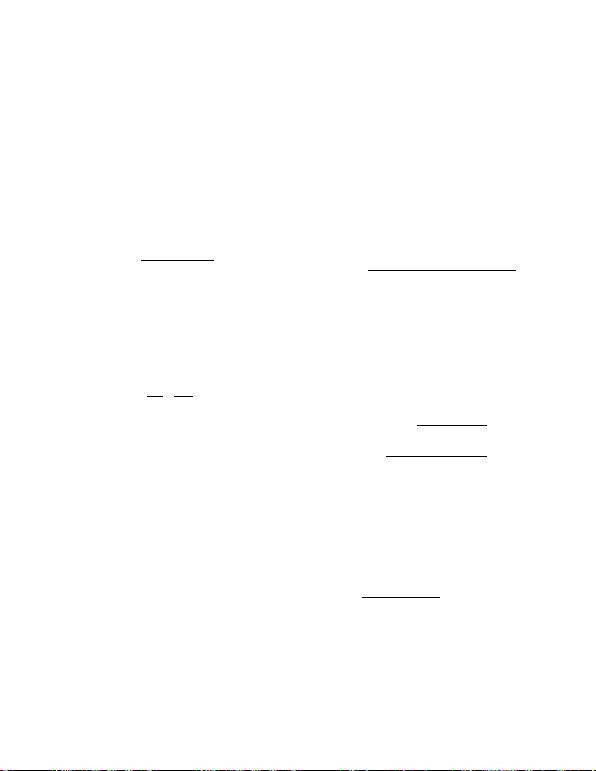An additional small LC filter may be necessary to sup-
press the remaining low-energy high-frequency spikes.
The LC filter also helps attenuate the switching frequen-
cy ripple. Care must be taken to avoid any compensa-
tion problems due to the insertion of the additional LC
filter. Design the LC filter with a corner frequency at more
than a decade higher than the estimated closed-loop,
unity-gain bandwidth to minimize its effect on the phase
margin. Use 1μF to 10μF low-ESR ceramic capacitors
and calculate the inductance using following equation:
where fC= estimated converter closed-loop unity-gain
frequency.
SEPIC Converter
The MAX15004A/B/MAX15005A/B can be configured
for SEPIC conversion when the output voltage must be
lower and higher than the input voltage when the input
voltage varies through the operating range. The duty-
cycle equation:
indicates that the output voltage is lower than the input
for a duty cycle lower than 0.5 while VOUT is higher
than the input at a duty cycle higher than 0.5. The
inherent advantage of the SEPIC topology over the
boost converter is a complete isolation of the output
from the source during a fault at the output. For the
MAX15004/MAX15005, the SEPIC converter output can
be fed back to VCC (Figure 6), so that the controller can
function even during cold-crank input voltage (2.5V).
Use a Schottky diode (DVIN) in the VIN path to avoid
backfeeding the input source. A current-limiting resistor
(RVCC) is also needed from the output to VCC depend-
ing upon the converter output voltage. The total VCC
current sink must be limited to 25mA. See the
Selecting
V
CC
Resistor (R
VCC
)
section to calculate the optimum
value of the VCC resistor.
tors, a MOSFET, series capacitance, and the rectifier
diode. The inductance is determined by the allowable
ripple current through all the components mentioned
above. Lower ripple current means lower peak and RMS
currents and lower losses. The higher inductance value
needed for a lower ripple current means a larger-sized
inductor, which is a more expensive solution. The induc-
tors L1 and L2 can be independent, however, winding
them on the same core reduces the ripple currents.
Calculate the maximum duty cycle using the following
equation and choose the RT and CT values accordingly
for a given switching frequency (see the
Oscillator
Frequency/External Synchronization
section).
where VDis the forward voltage of the Schottky diode,
VCS (0.305V) is the current-sense threshold of the
MAX15004/MAX15005, and VDS is the voltage drop
across the switching MOSFET during the on-time.
Inductor Selection in SEPIC Converter
Use the following equations to calculate the inductance
values. Assume both L1 and L2 are equal and that the
inductor ripple current (ΔIL) is equal to 20% of the input
current at nominal input voltage to calculate the induc-
tance value.
where fOUT is the converter switching frequency and η
is the targeted system efficiency. Use the coupled
inductors MSD-series from Coilcraft or PF0553-series
from Pulse Engineering, Inc. Make sure the inductor
saturating current rating (ISAT) is 30% higher than the
peak inductor current calculated using the following
equation. Use the current-sense resistor calculated
based on the ILPK value from the equation below (see
the
Current Limit
section).
IID
DII
LPK OUT MAX MAX
MAX OUT MAX L
=×
×++
()1ηΔ
LL L VD
fI
I
IN MIN MAX
OUT L
L
=== ×
××
=
122
02
Δ
Δ.×××
×
ID
D
OUT MAX MAX
MAX
()1η
DVV
VVVVV
MAX OUT D
IN MIN OUT D DS CS
=+
++ +
()
V
V
D
D
O
IN
=1
Lfc C
×××
1
410
32
4.5V to 40V Input Automotive
Flyback/Boost/SEPIC Power-Supply Controllers
MAX15004A/B/MAX15005A/B
20
Maxim Integrated